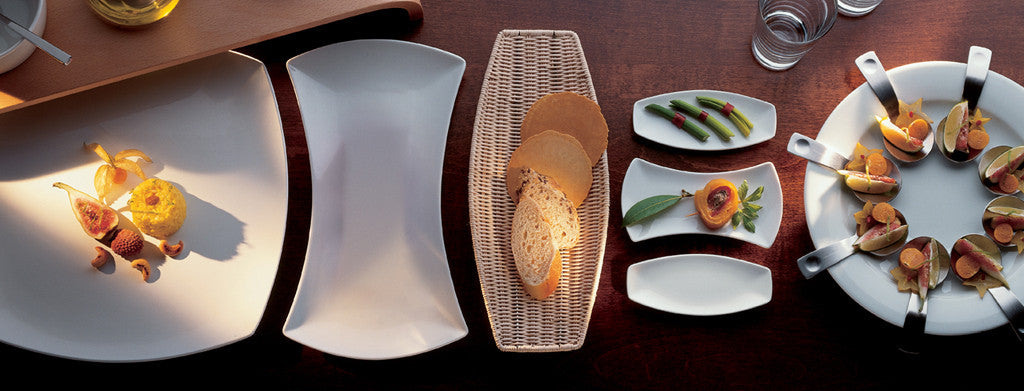Shopping Cart

Refine

View all

RangeCrockery£9.85 each (ex VAT)£9.85 each (ex VAT)£7.28 each (ex VAT)£8.18 each (ex VAT)£6.91 each (ex VAT)£5.93 each (ex VAT)£7.28 each (ex VAT)£1.10 each (ex VAT)£1.22 each (ex VAT)£5.22 each (ex VAT)£8.17 each (ex VAT)£6.30 each (ex VAT)£5.12 each (ex VAT)£8.18 each (ex VAT)£8.22 each (ex VAT)£5.43 each (ex VAT)£8.73 each (ex VAT)£5.32 each (ex VAT)£8.36 each (ex VAT)£6.04 each (ex VAT)£6.65 each (ex VAT)£8.22 each (ex VAT)£5.54 each (ex VAT)£7.08 each (ex VAT)£8.18 each (ex VAT)£6.12 each (ex VAT)£6.63 each (ex VAT)£9.03 each (ex VAT)£7.62 each (ex VAT)£5.45 each (ex VAT)£5.08 each (ex VAT)£5.36 each (ex VAT)£5.11 each (ex VAT)£5.93 each (ex VAT)£6.59 each (ex VAT)£5.30 each (ex VAT)£5.29 each (ex VAT)£5.73 each (ex VAT)£5.73 each (ex VAT)£8.83 each (ex VAT)£9.38 each (ex VAT)£6.65 each (ex VAT)£5.88 each (ex VAT)£6.30 each (ex VAT)£9.82 each (ex VAT)£7.14 each (ex VAT)£5.64 each (ex VAT)£5.77 each (ex VAT)